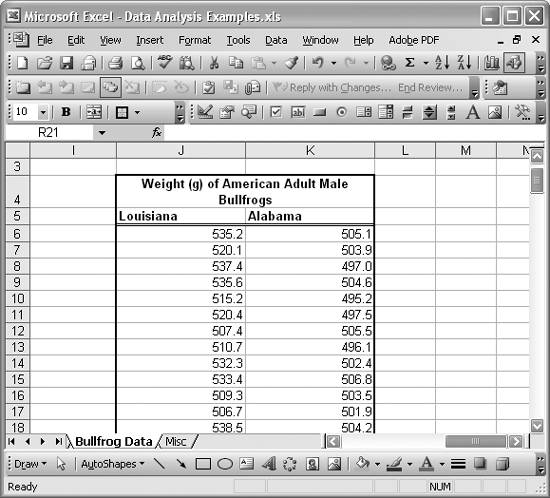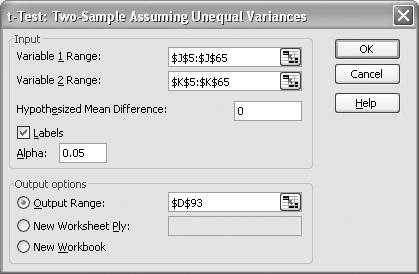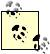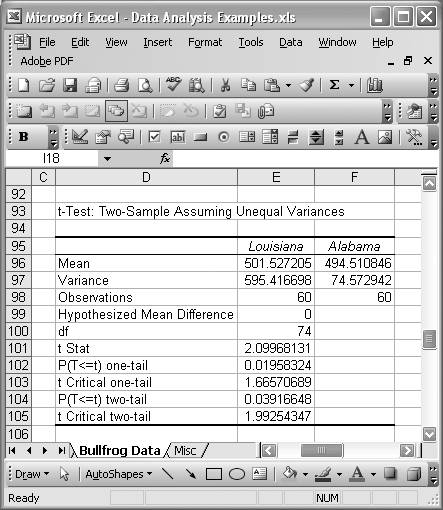# Performing Statistical Tests

Problem

You're engaged in hypothesis testing and you'd like to learn about Excel's support for conducting statistical tests .

Solution

Excel provides support for conducting standard z-tests, t-Tests, and F-tests. Read the following discussion to learn more.

Discussion

The built-in function ZTEST computes the one-tailed z-test using the normal distribution. The syntax for this function is =ZTEST(cell ref, m, s) where cell ref is a cell reference containing an input dataset, m is the value being tested, and s is the population standard deviation. If s is not known, it can be omitted, in which case the sample's standard deviation will be computed and used in the z-test calculation.

You can also compute two-tailed z-tests as follows: =2 * MIN(ZTEST(cell ref, m, s), 1 - ZTEST(cell ref, m, s)).

The function TTEST computes the one- or two-tailed t-test using Student's t-distribution. Further, TTEST allows you the option of performing paired, two sample with equal variance, or two sample with unequal variance tests. The syntax for TTEST is =TTEST(cell ref 1, cell ref 2, tails, type), where cell ref 1 and cell ref 2 are cell references to the two datasets under consideration and tails is the number of tails to consider for the test (1 or 2). type is a value of 1, 2, or 3, indicating the test type: paired, two sample equal variance, or two sample unequal variance, respectively.

FTEST computes the two-tailed F-test for two datasets. The syntax for FTEST is =FTEST(cell ref 1, cell ref 2), where cell ref 1 and cell ref 2 are cell references to the two datasets under consideration.

All three of these test functions have corresponding inverse functions (namely, NORMSINV, TINV, and FINV) that are useful when computing critical values for comparison. NORMSINV and TINV were discussed in Recipe 5.3. Recipe 8.7 makes use of FTEST and FINV while performing an F-test in the context of regression analysis.

The Analysis ToolPak also provides several tools for conducting statistical tests. These tools include:

• F-Test Two-Sample for Variances
• t-Test Paired Two-Sample for Means
• t-Test Two-Sample Assuming Equal Variances
• t-Test Two-Sample Assuming Unequal Variances
• z-Test Two-Sample for Means

To access these tools, select ToolsData Analysis from the main menu bar to open the Data Analysis dialog box. This is the same Data Analysis dialog box shown earlier in Figure 5-13. Youll find each of the statistical test tools listed in this dialog box. They all work pretty much the same way: select a tool to open the tool's dialog box, select input cell ranges, specify your hypothesis, and select your output options.

I'll show an example using the t-Test Two-Sample Assuming Unequal Variances tool. Figure 5-16 shows a portion of a spreadsheet containing measured weights of North American bullfrogs sampled from two southern states.

Each state's sample contains 60 data points. An example hypothesis to test here (a null hypothesis) is that there's no difference between the mean weights of bullfrogs from Louisiana and Alabama.

To conduct this test, select t-Test: Two-Sample Assuming Unequal Variances from the Data Analysis dialog box discussed earlier to open the t-Test dialog box shown in Figure 5-17.

Figure 5-16. Bullfrog weight dataFigure 5-17. t-Test dialog boxIn the Variable 1 Range and Variable 2 Range fields, type (or select from the spreadsheet) the cell ranges containing the dataset corresponding to Louisiana and Alabama, respectively. In this example, I included the column labels in these input ranges, so I checked the Labels checkbox.Alpha represents the level of significance related to the probability of encountering a type I error. 0.05 represents a 5% significant level. 0.01 represents a 1% significant level, and so on. See any standard text on statistics for more information on this value and hypothesis testing.

The hypothesis is that there is no difference between the means; therefore, enter a value of 0 in the Hypothesized Mean Difference field. In this example, I left the Alpha field (confidence field) at the default 5%; but you can change that as well. Finally, choose your output options. In this case, I chose to have the output on the same spreadsheet, starting at cell D93. Press OK; the results are shown in Figure 5-18.

Figure 5-18. t-Test resultsThese results include computed means and variances for the two input datasets, along with other information such as the number of observations, the hypothesized mean difference, and the degrees of freedom. The t-statistic is also reported, along with both the one-tailed and two-tailed probabilities and critical values. In this case, the t-statistic is 2.1, which is greater than the two-tailed t-critical value, thus indicating there is a statistically significant difference in means between the two datasets. You can also see that the P-value is less than the significance level (Alpha value of 5% in this case), which also indicates rejection of the null hypothesis.

The other statistical test tools available in the Analysis ToolPak generate results that look very much like the ones shown in Figure 5-18.Excel Scientific and Engineering Cookbook (Cookbooks (OReilly))
ISBN: 0596008791
EAN: 2147483647
Year: N/A
Pages: 206
Authors: David M Bourg